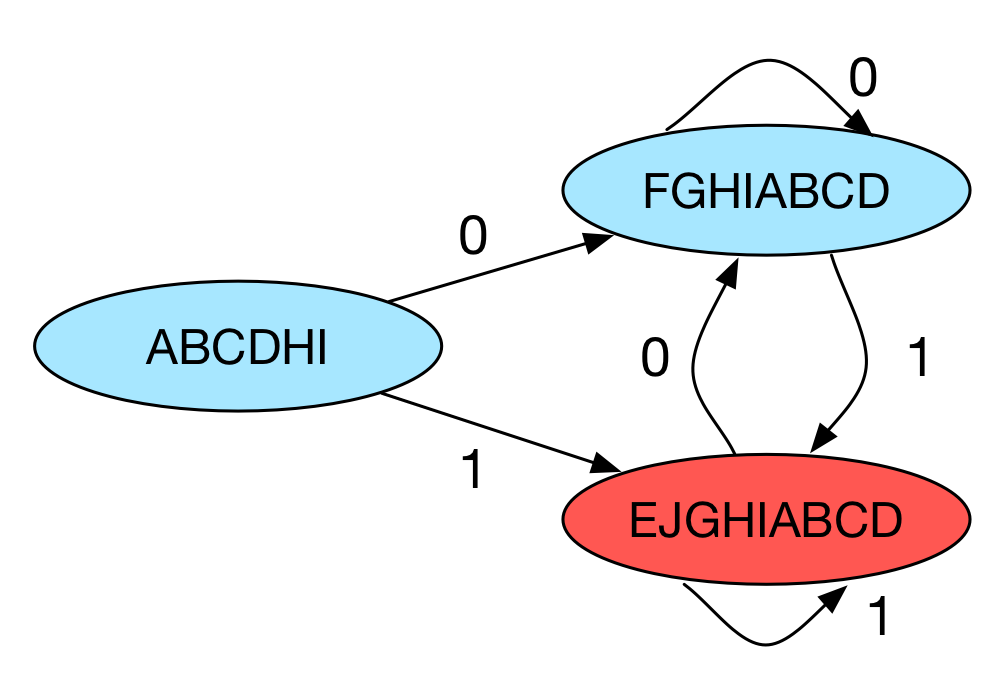# 编译原理之词法分析

Posted by YuanBao on April 19, 2016

## 编译型语言 VS 解释型语言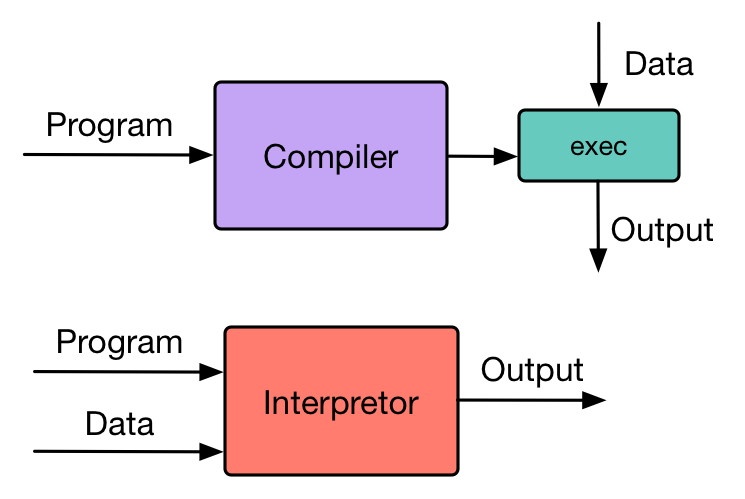图1：Compiler vs Interpretor

• 编译器读取用户使用某种语言编写的 源程序 ，并把改程序翻译成为一个等价的，用另一种语言编写的 目标程序 ，用户可以给定数据，并调用目标程序产生输出。
• 解释器并不通过翻译的方式生成目标程序。从用户的角度来看，解释器直接利用用户提供的输入来执行源程序文件中指定的操作。

## 编译的过程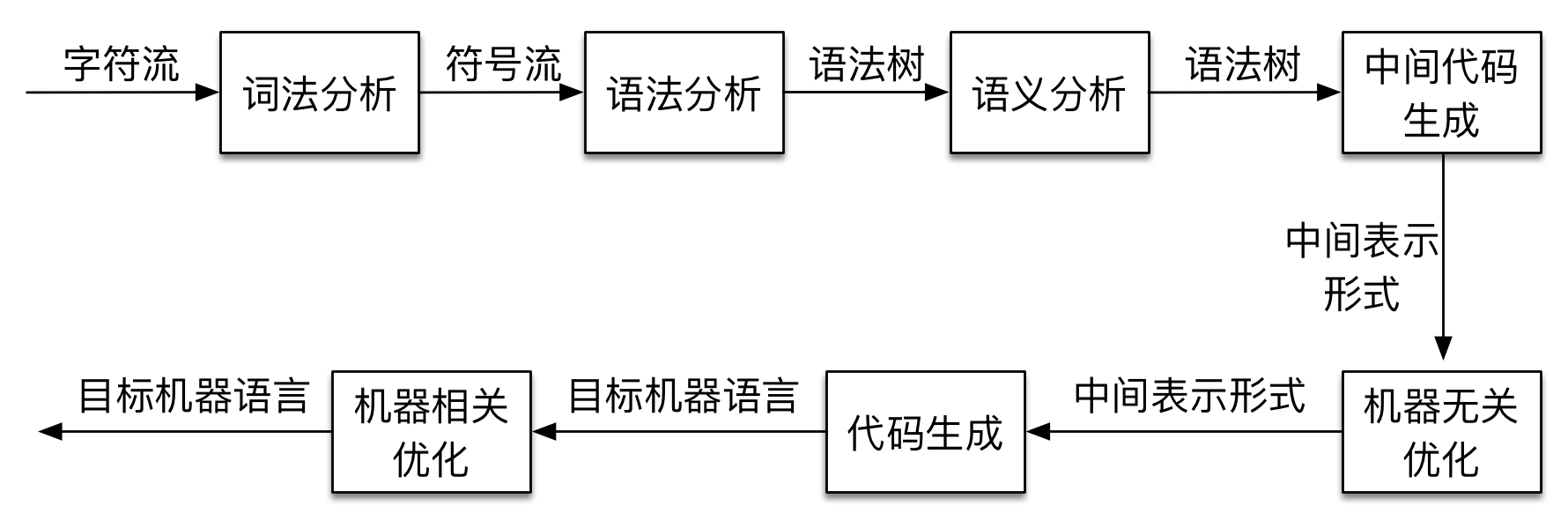图2：编译的过程

Compiler front-end optimizer generator
GCC GCC front-end GCC optimizer GCC code-generator
LLVM-gcc GCC front-end LLVM optimizer LLVM code-generator
Clang LLVM front-end LLVM optimizer LLVM code-generator

## 词法分析

1. 通过从左往右的读入源程序内容，将源程序划分成为一个个词素（注意每个词素都是一个字符串）。之后将根据不同的词素将其归为不同的词法单元（token）。词法分析中的 token 可以记为一个二元组 <token_class, token_value>, token 的种类比如标识符（Identifier），关键字（keyword），整数（integer），空白符（whitespace）等，token 的值即为词素。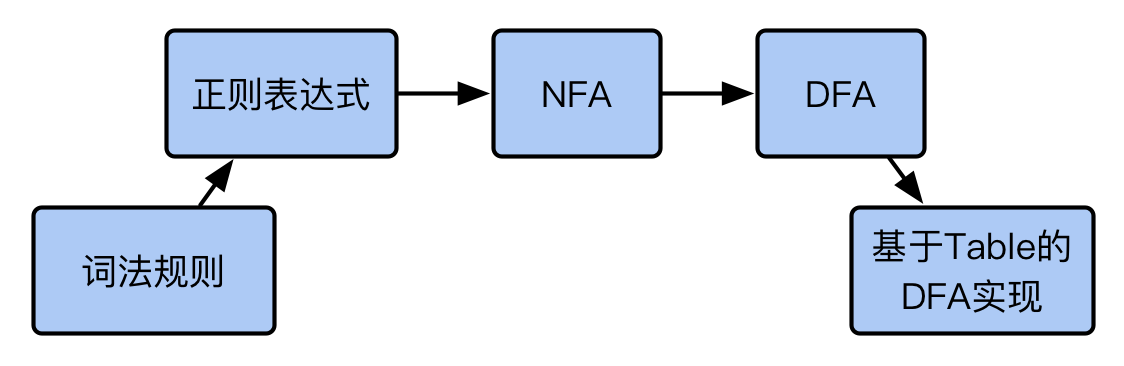图3：词法分析的基本步骤

#### 正则表达式

• 基本单元：任意单个字符 $c$ 组成的集合以及空字符 $\epsilon$ 集合.
• 基本操作：
• 合并（Union）：$A+B = \lbrace a \, \vert \, a \in A \rbrace \cup \lbrace b \, \vert \, b \in B \rbrace$
• 连接（Concatenation）：$AB = \lbrace ab \, \vert \, a \in A, b \in B \rbrace$
• 迭代（Iteration）：$A^{*} = {\cup}_{i \ge 0}A^{i}$

• 关键字 if 可以直接通过单个字母 if 通过连接操作而成；
• 任意长度的数字可以表示为 $[0\textit{-}9]^{*}$；
• 标识符可以表示为 $[ \_a\textit{-}zA\textit{-}Z][ \_a\textit{-}zA\textit{-}Z0\textit{-}9]^{*}$；

#### 自动机

1. 一个输入字符集 $\Sigma$
2. 一个有穷的状态集合 $\mathcal{S}$
3. 一个起始状态 $n$
4. 一个终止状态集合 $\mathcal{F} \subset \mathcal{S}$
5. 一系列状态转换 $state \stackrel{input}{\longrightarrow} state$

#### 正则语言到 NFA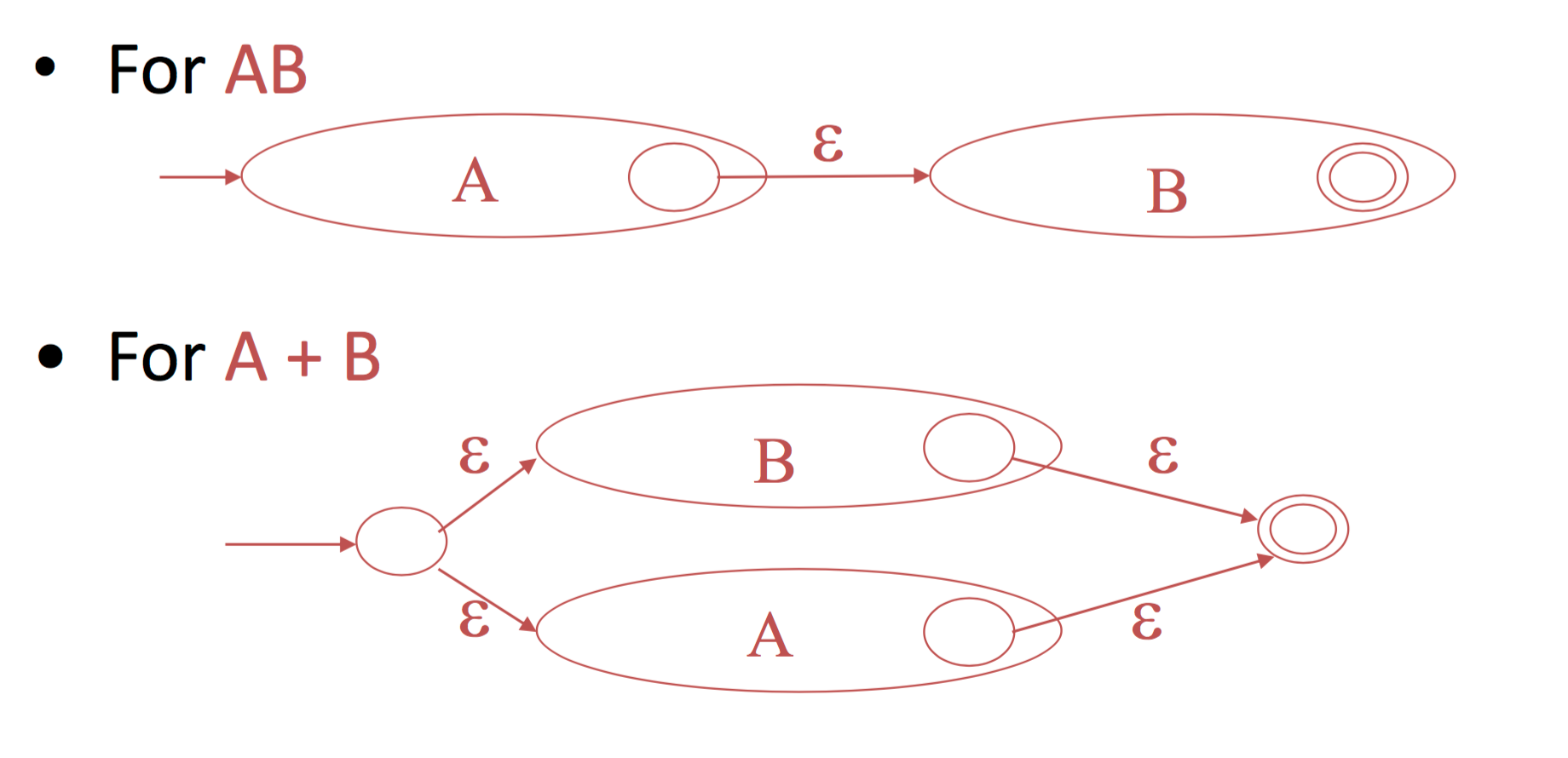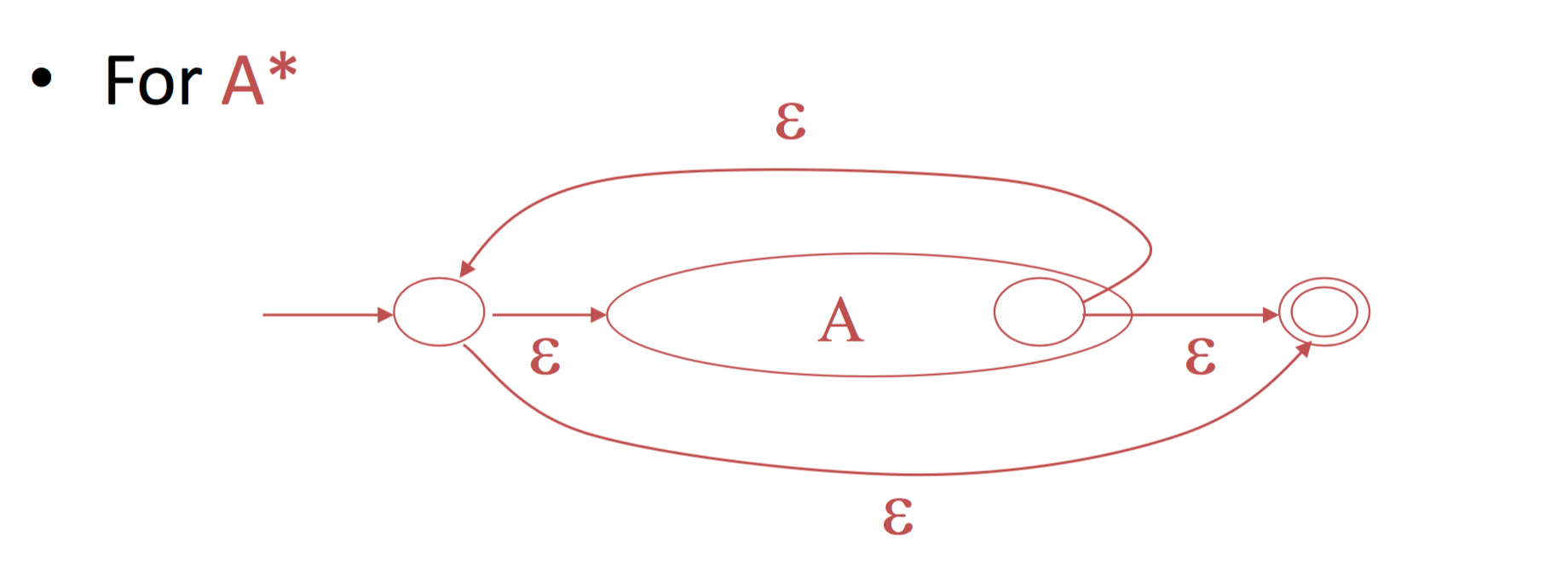图4：正则表达式生成 NFA

#### NFA 到 DFA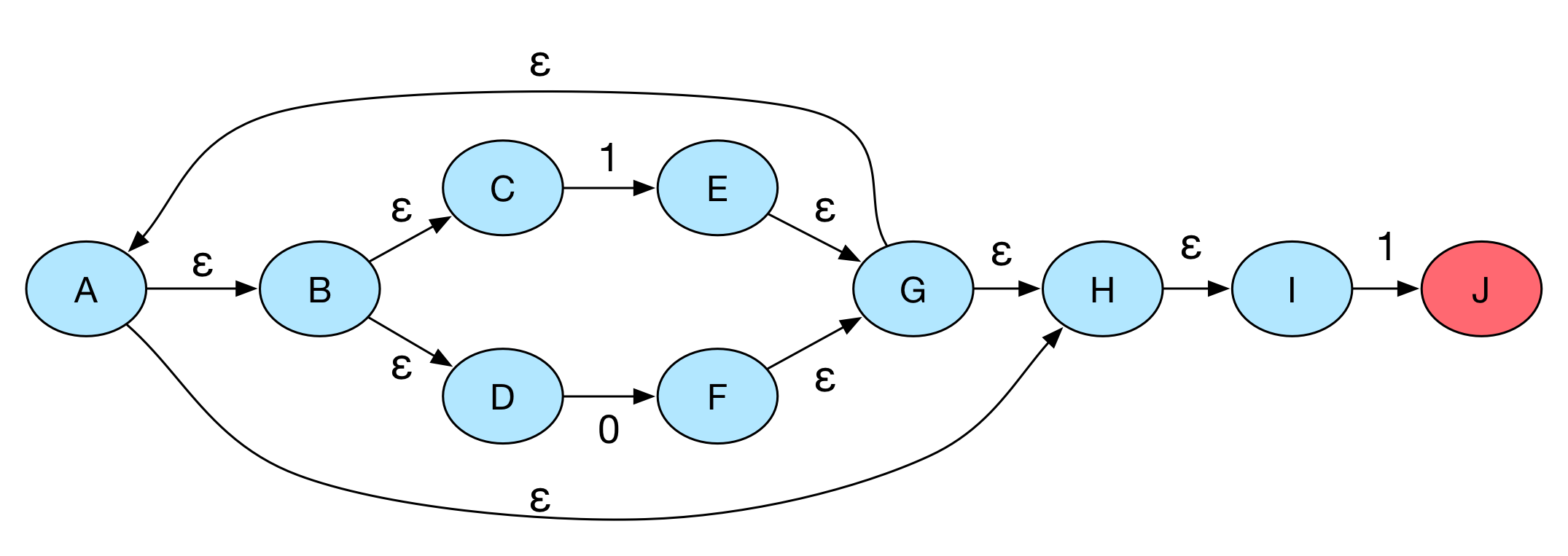• $\epsilon\textit{-}closure(B) = \lbrace B, C, D \rbrace$
• $\epsilon\textit{-}closure(G) = \lbrace A, B, C, D, G, H, I \rbrace$

• 状态集合：$subsets \, of \mathcal{S}$, $\mathcal{S}$ 是 NFA 的状态集合。
• 初始状态：$\epsilon\textit{-}closure(s)$，$s$ 是 NFA 的起始状态。
• 终止状态：$\lbrace X \, \vert \, X \wedge \mathcal{F} \ne \phi \rbrace$，其中$\mathcal{F}$是 NFA 的终止状态集合。
• 状态转换操作：$X \stackrel{a}{\rightarrow} Y \quad \textit{if} \quad Y = \epsilon\textit{-}closure(a(X))$。